Contents >> Applied Mathematics >> Numerical Methods >> Interpolation of Functions >> Newton’s second interpolation formula

 Interpolation of functions - Newton’s second interpolation formula Newton’s second interpolation formula Newton’s first interpolation formula is practically inconvenient for interpolation of functions near to end of the table. In this case usually Newton’s second interpolation formula is applied: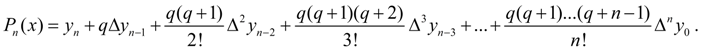(4) The detailed conclusion of the formula (4) is resulted in . Let's note, that if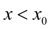and х it is close to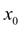it is meaningful to apply Newton’s first interpolation formula, if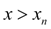and х it is close to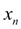, in this case is more convenient for using Newton’s second interpolation formula. In other words, Newton’s first interpolation formula is used usually for interpolation forward , and Newton’s second interpolation formula – for interpolation back .

 < Previous Contents Next >